## 10.2 – Quadratic Functions and their Graphs

### Learning Objectives

• (10.2.1) – Identify characteristics of a parabola
• vertex
• axis of symmetry
• $x$/$y$-intercepts
• General and standard forms of quadratic functions
• (10.2.2) – Classifying Solutions to Quadratic Equations

Curved antennas, such as the ones shown in the photo, are commonly used to focus microwaves and radio waves to transmit television and telephone signals, as well as satellite and spacecraft communication. The cross-section of the antenna is in the shape of a parabola, which can be described by a quadratic function.An array of satellite dishes. (credit: Matthew Colvin de Valle, Flickr)

# (10.2.1) – Identify characteristics of a parabola

The graph of a quadratic function is a U-shaped curve called a parabola. One important feature of the graph is that it has an extreme point, called the vertex. If the parabola opens up, the vertex represents the lowest point on the graph, or the minimum value of the quadratic function. If the parabola opens down, the vertex represents the highest point on the graph, or the maximum value. In either case, the vertex is a turning point on the graph. The graph is also symmetric with a vertical line drawn through the vertex, called the axis of symmetry.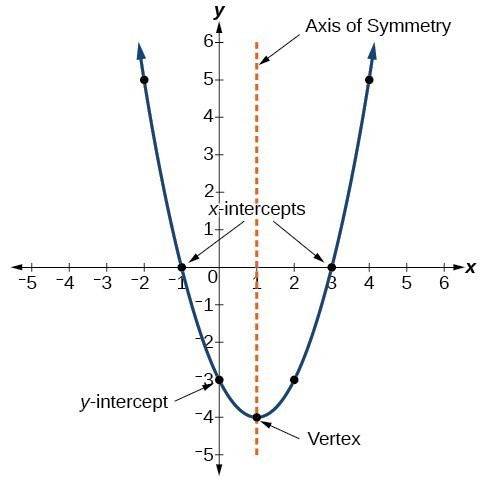The $y$-intercept is the point at which the parabola crosses the $y$-axis. The $x$-intercepts are the points at which the parabola crosses the $x$-axis. If they exist, the $x$-intercepts represent the zeros, or roots, of the quadratic function, the values of $x$ at which $y=0$.

### Example: Identifying the Characteristics of a Parabola

Determine the vertex, axis of symmetry, zeros, and y-intercept of the parabola shown below.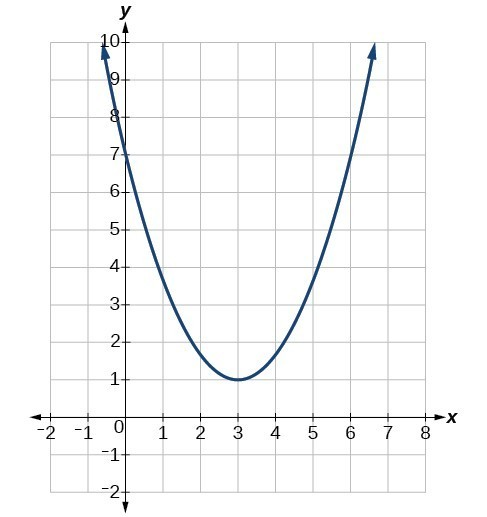### General and Standard Forms of Quadratic Functions

The general form of a quadratic function presents the function in the form

$f\left(x\right)=a{x}^{2}+bx+c$

where $a$, $b$, and $c$ are real numbers and $a\ne 0$. If $a>0$, the parabola opens upward. If $a<0$, the parabola opens downward. We can use the general form of a parabola to find the equation for the axis of symmetry.

The axis of symmetry is defined by $\displaystyle x=-\frac{b}{2a}$. If we use the quadratic formula, $\displaystyle x=\frac{-b\pm \sqrt{{b}^{2}-4ac}}{2a}$, to solve $a{x}^{2}+bx+c=0$ for the $x$-intercepts, or zeros, we find the value of $x$ halfway between them is always $\displaystyle x=-\frac{b}{2a}$, the equation for the axis of symmetry.

The figure below shows the graph of the quadratic function written in general form as $y={x}^{2}+4x+3$. In this form, $a=1,\text{ }b=4$, and $c=3$. Because $a>0$, the parabola opens upward. The axis of symmetry is $\displaystyle x=-\frac{4}{2\left(1\right)}=-2$. This also makes sense because we can see from the graph that the vertical line $x=-2$ divides the graph in half. The vertex always occurs along the axis of symmetry. For a parabola that opens upward, the vertex occurs at the lowest point on the graph, in this instance, $\left(-2,-1\right)$. The $x$-intercepts, those points where the parabola crosses the $x$-axis, occur at $\left(-3,0\right)$ and $\left(-1,0\right)$.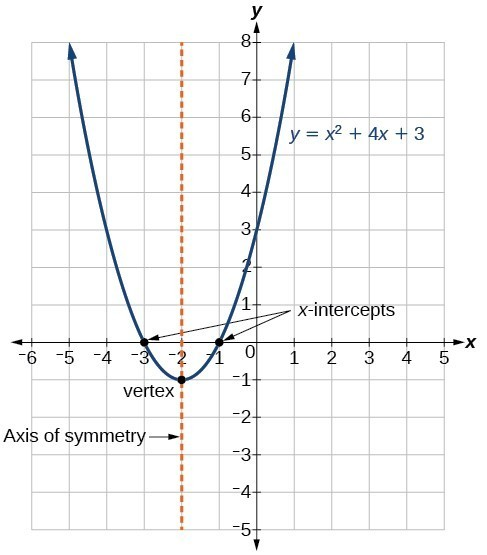The standard form of a quadratic function presents the function in the form

$f\left(x\right)=a{\left(x-h\right)}^{2}+k$

where $\left(h,\text{ }k\right)$ is the vertex. Because the vertex appears in the standard form of the quadratic function, this form is also known as the vertex form of a quadratic function.

### Given a quadratic function in general form, find the vertex of the parabola.

One reason we may want to identify the vertex of the parabola is that this point will inform us where the maximum or minimum value of the output occurs, ($k$), and where it occurs, ($x$). If we are given the general form of a quadratic function:

$f(x)=ax^2+bx+c$

We can define the vertex, $(h,k)$, by doing the following:

• Identify $a$, $b$, and $c$.
• Find $h$, the $x$-coordinate of the vertex, by substituting $a$ and $b$ into $\displaystyle h=-\frac{b}{2a}$.
• Find $k$, the $y$-coordinate of the vertex, by evaluating $\displaystyle k=f\left(h\right)=f\left(-\frac{b}{2a}\right)$

### Example: Finding the Vertex of a Quadratic Function

Find the vertex of the quadratic function $f\left(x\right)=2{x}^{2}-6x+7$. Rewrite the quadratic in standard form (vertex form).

### EXAMPLE

Given the equation $g\left(x\right)=13+{x}^{2}-6x$, write the equation in general form and then in standard form.

In this section, we will investigate quadratic functions further, including solving problems involving area and projectile motion. Working with quadratic functions can be less complex than working with higher degree polynomial functions, so they provide a good opportunity for a detailed study of function behavior.

# (10.2.2) – Classifying Solutions to Quadratic Equations

Much as we did in the application problems above, we also need to find intercepts of quadratic equations for graphing parabolas. Recall that we find the y-intercept of a quadratic by evaluating the function at an input of zero, and we find the x-intercepts at locations where the output is zero. Notice that the number of x-intercepts can vary depending upon the location of the graph.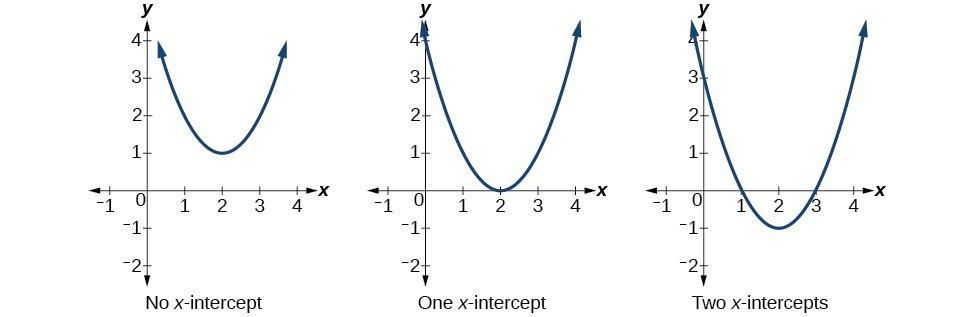Number of x-intercepts of a parabola

Mathematicians also define x-intercepts as roots of the quadratic function.

### How To: Given a quadratic function $f\left(x\right)$, find the $y$– and $x$-intercepts.

1. Evaluate $f\left(0\right)$ to find the $y$-intercept.
2. Solve the quadratic equation $f\left(x\right)=0$ to find the $x$-intercepts.

### Example: Finding the $y$– and $x$-Intercepts of a Parabola

Find the $y$– and $x$-intercepts of the quadratic $f\left(x\right)=3{x}^{2}+5x - 2$.

In the previous example, finding the $y$– and $x$-Intercepts of a parabola, the quadratic was easily solved by factoring. However, there are many quadratics that cannot be factored. We can solve these quadratics by first rewriting them in standard form.

### How To: Given a quadratic function, find the $x$-intercepts by rewriting in standard form.

1. Substitute $a$ and $b$ into $\displaystyle h=-\frac{b}{2a}$.
2. Substitute $x=h$ into the general form of the quadratic function to find $k$.
3. Rewrite the quadratic in standard form using $h$ and $k$.
4. Solve for when the output of the function will be zero to find the $x$intercepts.

### Example: Finding the Roots of a Parabola

Find the $x$-intercepts of the quadratic function $f\left(x\right)=2{x}^{2}+4x - 4$.

### EXAMPLE

Find the $y$-intercept for the function $g\left(x\right)=13+{x}^{2}-6x$.

#### Complex Roots

Now you will hopefully begin to understand why we introduced complex numbers at the beginning of this module. Consider the following function: $f(x)=x^2+2x+3$, and it’s graph below: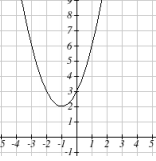Does this function have roots? It’s probably obvious that this function does not cross the $x$-axis, therefore it doesn’t have any $x$-intercepts. Recall that the $x$-intercepts of a function are found by setting the function equal to zero:

$x^2+2x+3=0$

In the next example, we will solve this equation.  You will see that there are roots, but they are not $x$-intercepts because the function does not contain $(x,y)$ pairs that are on the $x$-axis.  We call these complex roots.

By setting the function equal to zero and using the quadratic formula to solve, you will see that the roots contain complex numbers:

$x^2+2x+3=0$

### Example

Find the $x$-intercepts of the quadratic function. $f(x)=x^2+2x+3$

#### Important Terms

axis of symmetry
a vertical line drawn through the vertex of a parabola around which the parabola is symmetric; it is defined by $\displaystyle x=-\frac{b}{2a}$.
general form of a quadratic function
the function that describes a parabola, written in the form $f\left(x\right)=a{x}^{2}+bx+c$, where $a$, $b$, and $c$ are real numbers and $a\ne 0$.
standard form of a quadratic function
the function that describes a parabola, written in the form $f\left(x\right)=a{\left(x-h\right)}^{2}+k$, where $\left(h,\text{ }k\right)$ is the vertex.
vertex
the point at which a parabola changes direction, corresponding to the minimum or maximum value of the quadratic function
zeros
in a given function, the values of $x$ at which $y = 0$, also called roots